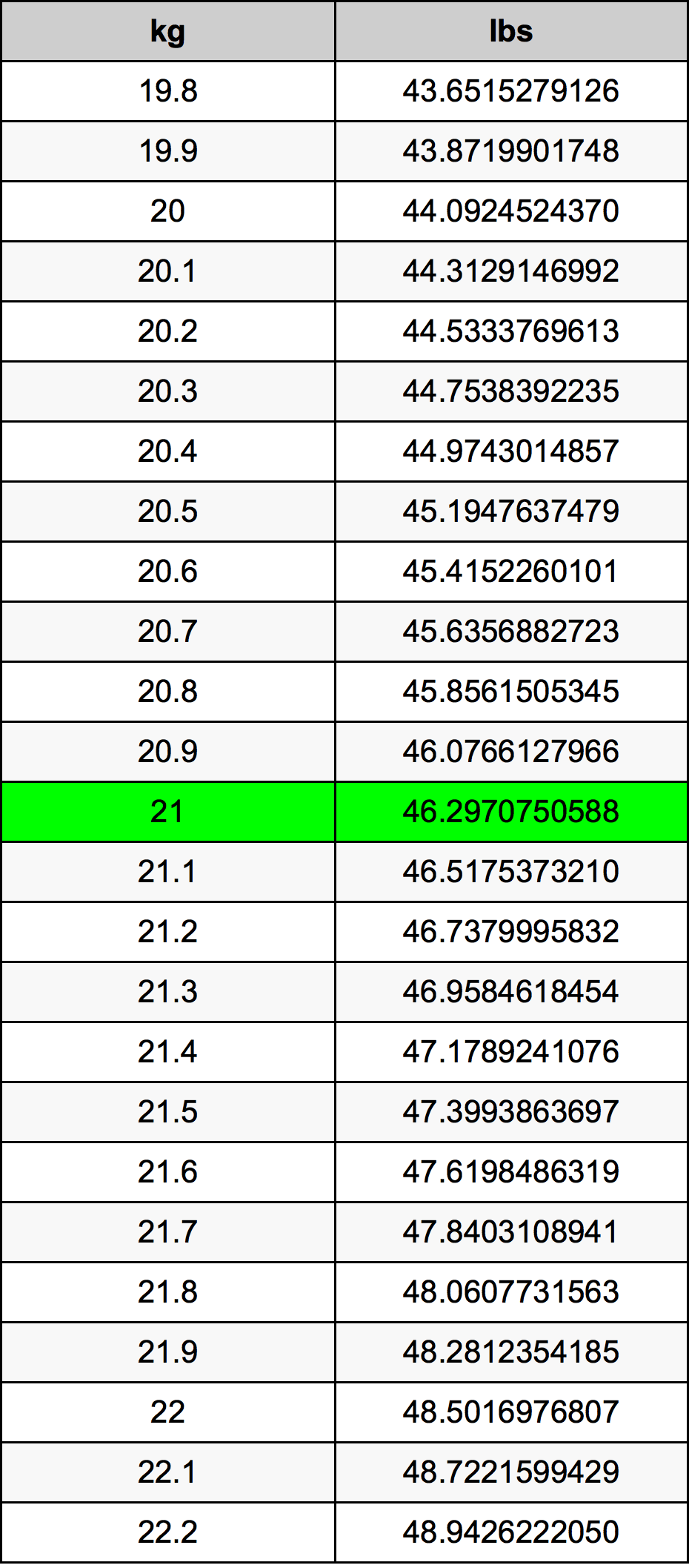Kg To Lbs

# 21 kg to lbs21 Kilograms to Pounds

kg
=
lbs

## How to convert 21 kilograms to pounds?

 21 kg * 2.2046226218 lbs = 46.2970750588 lbs 1 kg
A common question is How many kilogram in 21 pound? And the answer is 9.52543977 kg in 21 lbs. Likewise the question how many pound in 21 kilogram has the answer of 46.2970750588 lbs in 21 kg.

## How much are 21 kilograms in pounds?

21 kilograms equal 46.2970750588 pounds (21kg = 46.2970750588lbs). Converting 21 kg to lb is easy. Simply use our calculator above, or apply the formula to change the length 21 kg to lbs.

## Convert 21 kg to common mass

UnitMass
Microgram21000000000.0 µg
Milligram21000000.0 mg
Gram21000.0 g
Ounce740.753200941 oz
Pound46.2970750588 lbs
Kilogram21.0 kg
Stone3.3069339328 st
US ton0.0231485375 ton
Tonne0.021 t
Imperial ton0.0206683371 Long tons

## What is 21 kilograms in lbs?

To convert 21 kg to lbs multiply the mass in kilograms by 2.2046226218. The 21 kg in lbs formula is [lb] = 21 * 2.2046226218. Thus, for 21 kilograms in pound we get 46.2970750588 lbs.

## 21 Kilogram Conversion Table## Alternative spelling

21 Kilogram to Pound, 21 Kilogram in Pound, 21 kg to lbs, 21 kg in lbs, 21 Kilogram to lbs, 21 Kilogram in lbs, 21 Kilograms to Pound, 21 Kilograms in Pound, 21 Kilograms to Pounds, 21 Kilograms in Pounds, 21 Kilogram to Pounds, 21 Kilogram in Pounds, 21 Kilograms to lbs, 21 Kilograms in lbs, 21 kg to Pound, 21 kg in Pound, 21 Kilogram to lb, 21 Kilogram in lb